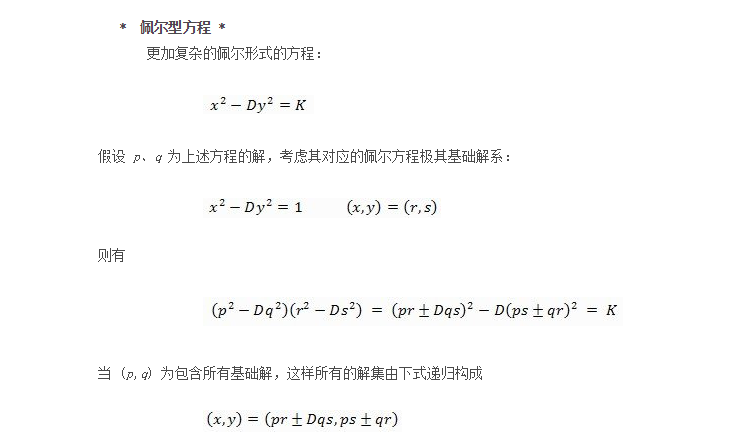# 佩尔方程实例讲解

hresh 629## 前言

x^2-d*y^2= 1 ........①x^2-d*y^2= -1 ........②

x+y√d=(a+b√d)^(2n+1) （n为任意正整数）## 实例分析

### 案例一

记得有一次全班去唱K, 其中有个活动是情歌对唱. 具体操作流程是这样的:



x(n+1) = 3xn±4yn
y(n+1) = 2xn±3yn

#由于题目解要求为正整数且 x(n+1) 大于 xn，（1，1）为方程基本解，因此选择乘积相加，而非相减。
x(n+1) = 3xn+4yn
y(n+1) = 2xn+3yn


N = 54
def cal_probability2():
global N

x = 1
y = 1
n = 0

xy_list = []
while (x + 1) / 2 <= N:
a = x
b = y
if x>y:
xy_list.append(((x + 1) // 2, (y + 1) // 2))
n += 1
x = 3 * a + 4 * b
y = 2 * a + 3 * b
print(n)
print(xy_list)


2
[(4, 3), (21, 15)]


### 案例二

数1,3,6,10,....构成数列{a_n}，数1,4,9,16,....构成数列{b_n}。将既是数列{a_n}中的项，又是数列{b_n}中的项按照从小到大的顺序



N = 4

n = 1
a = 3   #方程最小解(x1,y1)
b = 1

nm_list = []
while n<=4:
x = a
y = b
nm_list.append(((x-1)//2,y))
a = 3*x+8*y
b = x+y*3
n += 1

print(nm_list)

result = []
for w in nm_list:
b_n = w**2
result.append(b_n)
print(result)


[(1, 1), (8, 6), (49, 35), (288, 204)]
[1, 36, 1225, 41616]